Quantity: 0

Total: 0,00

# Cube sections (exercise)

### Cube sections (exercise)

Examining solids formed by the intersection of a cube and a plane.

Mathematics

Keywords

cube, Euler's Theorem, section, polyhedron, solids, vertex, edge, face, 3D, mathematics

### Related items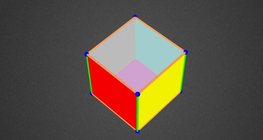#### Coloring a cube

Coloring the vertices, edges and faces of a given cube according to the criteria specified in the exercise.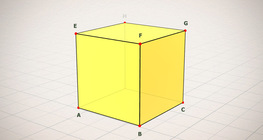#### Cube (exercises)

Edges, diagonals and faces of a cube can be identified by its vertices.#### Cube of cubes

An exercise about the regular hexahedron built from unit cubes to help deepen your knowledge of cubes.#### Cube puzzle

Building cubes shown in several views from the available unit cubes aids spatial vision and other skills.#### Net of a cube (exercises)

Not all nets consisting of 6 congruent squares are foldable into cubes.#### Cube

This animation demonstrates the components (vertices, edges, diagonals and faces) of the cube, one of the Platonic solids.#### Cuboid

A cuboid is a polyhedron with six rectangular faces.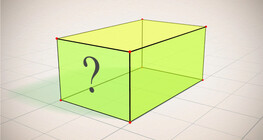#### Cuboid (exercises)

Edges, diagonals and faces of a cuboid can be identified by its vertices.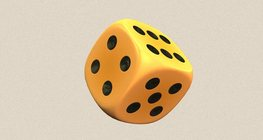#### Dice

Regular dice can be used for solving statistical and probability exercises.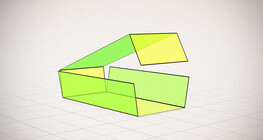#### Nets of a cuboid (exercises)

This animation demonstrates the different nets of a cuboid and includes a game.#### Notable products

A spectacular demonstration of algebraic expressions.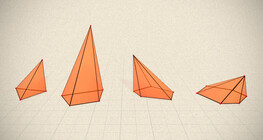#### Regular square pyramid

A regular square pyramid is a right pyramid with a square base and four triangular faces.#### Volume and surface area (exercise)

An exercise about the volume and surface area of solids generated from a ´base cube´.#### Optical illusion

The information gathered by the eye is misinterpreted by the brain.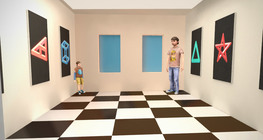#### Ames room

An Ames room is a distorted room that is used to create an optical illusion.